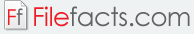### StatPlus 2009 Professional 5.7.8

#### AnalystSoft (Shareware)

StatPlus 2009 Professional is an application that assists users in processing statistical data. It comes with a comprehensive set of tools and functions not only for statistics use but for graphical analysis as well. There are over 90 built-in functions for financial analysis, mathematical calculations, and statistical data processing, all with familiar Excel names. This application comes with an intuitive and straightforward interface that makes it easy for users to access the program’s tools. Some of its statistical features are the following:

• Basic Statistics – frequency tables analysis, normality tests, z-tests
• Nonparametric Statistics – Cochran Q Test, Chi-square test, 2x2 table analysis
• Analysis of Variance / ANOVA – general linear models, and up to three-way analysis
• Regression Analysis – logistic, stepwise, polynomial, multivariate linear regression analysis
• Time Series Analysis – Fourier analysis, moving average, interrupted time series

Among the data processing functions included are transformations, random number generation, matrix operations, sampling, and standardization. StatPlus 2009 Professional also enables users to create different kinds of statistical charts, including histograms, point-graphs, control charts, and pie charts. Users can also process data for survival analysis, forecasting, and time series analysis. Users can export and import spreadsheets to and from various statistical programs such as Microsoft Excel, StatSoft Statistica, and SPSS Documents. This application is also equipped with a spell checker.